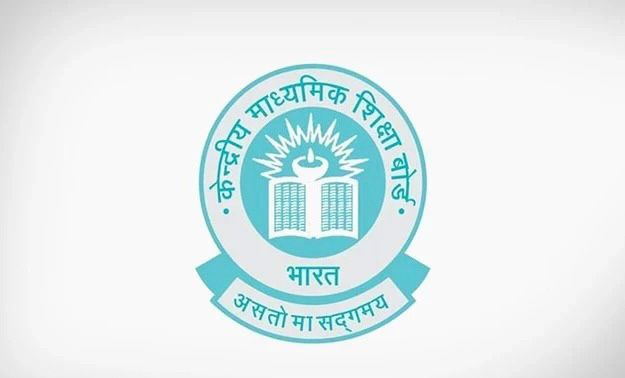# Three vertices of a parallelogram ABCD are A(1, 4), B(-2, 3) and C(5,8). The ordinate of the fourth vertex D is

CBSE Maths Exam 2021-22 Term 1, question: Three vertices of a parallelogram ABCD are A(1, 4), B(-2, 3) and C(5,8). The ordinate of the fourth vertex D isa) 8

b) 9

c) 7

d) 6